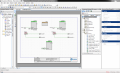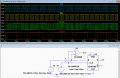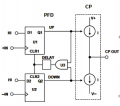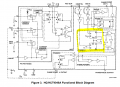# Fix pulse width, add and subtract frequencies

#### Eshawn

Joined Oct 5, 2019
31
Hello everyone:

Suppose we have two digital input frequencies in1 and in2 in the range of about a little less than or greater than 100 kHz each.

My objective is to add and subtract the two input frequencies to get an output frequency which is equal to out = in1 + in2 Hz or out = in1 - in2 Hz. I imagine that a prerequisite to get the right output is that both in1 and in2 must have equal pulse although in1 and in2 May have very different frequencies.

So:

1- if it’s is necessary to fix the pulse width, is there a trivial solution preferably a CMOS solution to fix the pulse width of one of the input frequencies to the pulse width of the other input frequency?

2- is there a trivial solution to get an output frequency which is the sum or the different of the two input frequencies?

Thank you

#### crutschow

Joined Mar 14, 2008
26,991
I think an XOR gate will give the difference frequency if both signals are a square-wave (50% duty-cycle), and the sum frequency if the pulse-widths are short.
Running the signal through a toggle flip-flop gives a 50% duty-cycle (but giving 1/2 the frequency).
Running it through a one-shot can generate a short pulse-width.

Joined Mar 10, 2018
4,057
Do you need this to be an instantaneous sum or difference, or can there
be latency to producing the correct result.

Asking because you could do two freq cntrs in a PSOC and gen the result with
a DDS.In fact one could add the wavedac component, use the DDS to drive it, and generate
the result as a sine, saw, tri, arbitrary waveshape.

If you source signals sinusoidal easy to add two onchip comparators to design
to get clean digital signals to compare.

All on one chip.

Regards, Dana.

Last edited:

Joined Mar 10, 2018
4,057

#### WBahn

Joined Mar 31, 2012
26,398
Hello everyone:

Suppose we have two digital input frequencies in1 and in2 in the range of about a little less than or greater than 100 kHz each.

My objective is to add and subtract the two input frequencies to get an output frequency which is equal to out = in1 + in2 Hz or out = in1 - in2 Hz. I imagine that a prerequisite to get the right output is that both in1 and in2 must have equal pulse although in1 and in2 May have very different frequencies.

So:

1- if it’s is necessary to fix the pulse width, is there a trivial solution preferably a CMOS solution to fix the pulse width of one of the input frequencies to the pulse width of the other input frequency?

2- is there a trivial solution to get an output frequency which is the sum or the different of the two input frequencies?

Thank you
It depends on some details that are lacking.

For instance, what do these input signals look like. Are they fixed-duty cycle? Fixed-pulse width? Are they largely random signals?

What does the output need to look like? Does the shape of the waveform need to look anything like the shape of one or the other input signals?

Imagine two 50% duty cycle input signals at 100 kHz that are perfectly aligned (for instance, imagine simply taking the same signal and applying it to your two input ports). Do you need the output to be a 50% duty cycle signal at 200 kHz (for the sum signal) and a DC signal for the difference signal? What should the DC signal be? HI or LO?

Now imagine passing one of those signals through an inverter to generate the other signal. What, if any, difference should there be in your output?

How about if the signal is passed through a delay element so that it is 90° out of phase with the first signal. What, if any, difference should there be in your output?

#### Eshawn

Joined Oct 5, 2019
31
Do you need this to be an instantaneous sum or difference, or can there
be latency to producing the correct result.

Asking because you could do two freq cntrs in a PSOC and gen the result with
a DDS.

View attachment 187364

In fact one could add the wavedac component, use the DDS to drive it, and generate
the result as a sine, saw, tri, arbitrary waveshape.

If you source signals sinusoidal easy to add two onchip comparators to design
to get clean digital signals to compare.

All on one chip.

Regards, Dana.

#### Eshawn

Joined Oct 5, 2019
31
Thanks for all responses.
In response to your question Dana, I would rather to do the addition and subtraction directly on the frequencies without using a counter. That is why I also raised the question if pulse width.

#### crutschow

Joined Mar 14, 2008
26,991
I was mistaken about an XOR gate giving the difference frequency but it can generate the sum frequency.

Below is the LTspice simulation of a circuit using a D-FF to give the difference frequency, and an XOR gate to give the sum.
Both are independent of the pulse duty-cycle.
It shows the 10 kHz difference frequency between inputs of 100 kHz and 90 kHz and the 190 kHz sum frequency.#### Eshawn

Joined Oct 5, 2019
31
Thank you Crutschow.
This is a very interesting solution that is also independent of duty cycle.
Would it be possible to explain how the difference of duty cycle of the two input frequencies cannot cause any error in the output frequency or how the two input pulses with different pulse width can neatly align?

Thank you

#### crutschow

Joined Mar 14, 2008
26,991
Would it be possible to explain how the difference of duty cycle of the two input frequencies cannot cause any error in the output frequency or how the two input pulses with different pulse width can neatly align?
Both circuits work on the edge change so duty-cycle is not a factor in the accuracy.
Edit: That is not accurate. It's best if the duty-cycle is near 50%.

There could be an error when the two edges are closely aligned, which can cause stump spikes out of the XOR gate, or a quasi-stable state of the flip-flop.
Don't know if those will cause unacceptable errors in your application.What accuracy do you require?

Last edited:

#### Eshawn

Joined Oct 5, 2019
31
Thank you.
An error margin of 1 to 3 % could be acceptable. What percentage of error do you think could happen with the suggested solution?
Also what would the results of subtraction in1 - in2 be if the in1 has lower frequency Hz than the in2? And in particular what would be the result of the subtraction if in1 frequency stops and has zero Hz?

#### Tesla23

Joined May 10, 2009
439
Have you thought about a phase frequency detector:the two clock signals are fed to the +IN and -IN inputs. This one is configured to feed a charge pump, but for your application a voltage output is probably simpler. There is one in the CMOS 4046 PLL chip which may be suitable for you - it is Phase Comparitor 2. See http://www.ti.com/lit/an/scha003b/scha003b.pdf:If my memory serves me correctly, feeding your signals into COMP IN and SIG IN, and low pass filtering Phase Comp II Output (pin 13), you will get a signal that has a DC component that changes from 0.25Vcc to 0.75Vcc depending whether the freq 1 is above or below freq 2, and superimposed on this is a sawtooth at the difference frequency with amplitude 0.5Vcc. All this comes out as something resembling PWM at your 100kHz comparison frequency.

#### crutschow

Joined Mar 14, 2008
26,991
What percentage of error do you think could happen with the suggested solution?
Hard to say.
I think it would be less than 3% but you probably need to build the circuit to determine that.
Also what would the results of subtraction in1 - in2 be if the in1 has lower frequency Hz than the in2?
Makes no difference.
what would be the result of the subtraction if in1 frequency stops and has zero Hz?
The difference output would be stuck at zero or one, depending upon which input stops and when.
If the CLK input stops, the Dif output will stay at the last value before the stop.
If the D input stops, then the Dif output will stay in the same state that the D input is when it stops.

#### WBahn

Joined Mar 31, 2012
26,398
I was mistaken about an XOR gate giving the difference frequency but it can generate the sum frequency.

Below is the LTspice simulation of a circuit using a D-FF to give the difference frequency, and an XOR gate to give the sum.
Both are independent of the pulse duty-cycle.
It shows the 10 kHz difference frequency between inputs of 100 kHz and 90 kHz and the 190 kHz sum frequency.
View attachment 187367
So you are saying that if the same signal is applied both inputs of an XOR gate that the result will be a signal at twice the frequency?

Are you also saying that if I apply a 10 Hz signal to the clock of a DFF and a 100 kHz signal to the D input that the output will be a signal at 90 kHz?

#### crutschow

Joined Mar 14, 2008
26,991
So you are saying that if the same signal is applied both inputs of an XOR gate that the result will be a signal at twice the frequency?
Not if they are identical in phase and pulse width.
Are you also saying that if I apply a 10 Hz signal to the clock of a DFF and a 100 kHz signal to the D input that the output will be a signal at 90 kHz?
No.
It only works if the two frequencies are close, as the TS needs.
Not sure of the maximum but it works with differences of 10kHz or less.

Joined Mar 10, 2018
4,057
Thank you.
An error margin of 1 to 3 % could be acceptable. What percentage of error do you think could happen with the suggested solution?
Also what would the results of subtraction in1 - in2 be if the in1 has lower frequency Hz than the in2? And in particular what would be the result of the subtraction if in1 frequency stops and has zero Hz?
This can all be handled digitally and produce correct outputs. Negative differential frequency could
be handled either as 0 Hertz out or as absolute difference (which would yield always a + freq).

Error easily << 1% if done with xtal control, maybe 2% no xtal.

If freq in is 0 hertz output could be 0 hertz or whatever you want.

But this requires a Micro. Latency would be gate time of freq cntr, or
if using reciprocal counting the 100 Khz period.

Regards, Dana.

#### Eshawn

Joined Oct 5, 2019
31
This can all be handled digitally and produce correct outputs. Negative differential frequency could
be handled either as 0 Hertz out or as absolute difference (which would yield always a + freq).

Error easily << 1% if done with xtal control, maybe 2% no xtal.

If freq in is 0 hertz output could be 0 hertz or whatever you want.

But this requires a Micro. Latency would be gate time of freq cntr, or
if using reciprocal counting the 100 Khz period.

Regards, Dana.

#### Eshawn

Joined Oct 5, 2019
31
Thank you for your comment Dana.

I had already implemented the digital solution for this. I started this thread to see if adding and subtracting the frequencies directly would allow a more trivial solution. But after all responses it appears to me that the digital solution is the simplest and easiest way of implementing it.

But I am not sure what you meant by the ‘micro’ and ‘reciprocal counting’ in your last paragraph.
Could you elaborate that?

Joined Mar 10, 2018
4,057
Micro, using a processor to implement two freq counters, and then a DDS to generate
the sum or difference. This can be done on one chip. Including analog to clean up
signals.

Reciprocal counting is a technique in frequency counters to handle super low frequencies.

http://leapsecond.com/hpan/an200.pdf

Regards, Dana.

Joined Mar 10, 2018
4,057
Here is a possible solution, one chip. Analog and Digital all
on the PSOC.

Comparator is used to condition freq inputs, if needed.

Mux is used and each channel period is measure, then code programs the SumDDS and DiffDDS
with appropriate freq words.

Right hand window shows resources used. As you can see quite a lot of resources leftThere is enough resources to implement two freq cntrs and do it totally in HW so
code only reads the current freqs and programs the DDS's. Might be even possible
to use DMA and the internal DataPath tool to do everything in HW thereby minimizing
latency from a freq change to the sum and diff DDS updates.

Also if needed could use the WaveDAC component, instantiate two in the design,
and have the DDS sum and diff outputs clock them to produce sine out of sum and
diff, or any other arbitrary waveform. See attached. There are enough resources onchip
to do all this.

Regards, Dana.

Last edited: